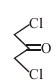$\stackrel{\mathrm{HCN}}{\to }$A $\underset{\left(excess\right)}{\overset{KCN}{\to }}$B;B is

(1)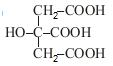(2)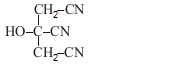(3)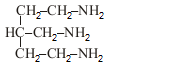(4)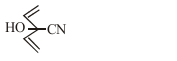Concept Questions :-

Acid Derivatives, Preparation and Properties
High Yielding Test Series + Question Bank - NEET 2020

Difficulty Level: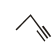$\stackrel{{\mathrm{Hg}}^{2+}/{\mathrm{H}}_{2}{\mathrm{SO}}_{4}}{\to }$Product;

(1)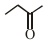(2)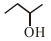(3)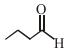(4)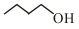High Yielding Test Series + Question Bank - NEET 2020

Difficulty Level: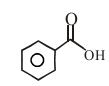$\underset{∆}{\overset{{\mathrm{P}}_{2}{\mathrm{O}}_{5}}{\to }}$Product

Product is?

(1)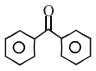(2)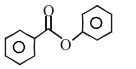(3)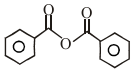(4)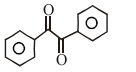Concept Questions :-

Preparation & properties of Aldehydes and Ketones
High Yielding Test Series + Question Bank - NEET 2020

Difficulty Level: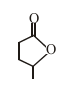Product,

Product is:-

1.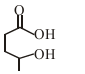2.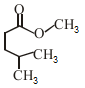3.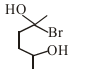4.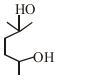Concept Questions :-

Preparation & properties of Aldehydes and Ketones
High Yielding Test Series + Question Bank - NEET 2020

Difficulty Level: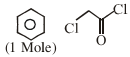$\stackrel{{\mathrm{FeCl}}_{3}}{\to }$ Product(Major):-

(1)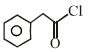(2)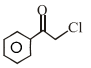(3)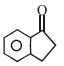(4)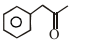Concept Questions :-

Nucleophillic Addition, Reactivity of Alpha Hydrogen in Aldehydes with Uses
High Yielding Test Series + Question Bank - NEET 2020

Difficulty Level:

Which of the following is correct matched

(a)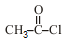$\stackrel{\mathrm{Pd}-{\mathrm{BaSO}}_{4}}{\to }$Rosenmunds Reduction

(b)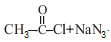$\to$Curtius Reaction

(c)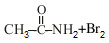$\stackrel{\mathrm{KOH}}{\to }$ Hoffmann's bromamide reaction

(d)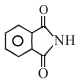$\underset{{\mathrm{CH}}_{3}-\mathrm{Cl}}{\overset{\mathrm{KOH}}{\to }}$ Gabriel pthalimide reaction

(1) a,d

(2) a,b,d

(3) b,c,d

(4) All

Concept Questions :-

Preparation & properties of Aldehydes and Ketones
High Yielding Test Series + Question Bank - NEET 2020

Difficulty Level: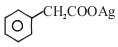+Br2 $\underset{∆}{\overset{{\mathrm{CCl}}_{4}}{\to }}$?

1.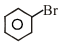2.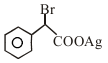3.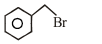4.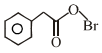Concept Questions :-

Carboxylic Acids
High Yielding Test Series + Question Bank - NEET 2020

Difficulty Level:

Which of the following acid is most easily decarboxylate :-

(1)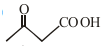(2)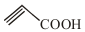(3)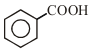(4)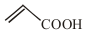Concept Questions :-

Carboxylic Acids
High Yielding Test Series + Question Bank - NEET 2020

Difficulty Level:

Identify the product (c) in the series

CH3CN$\stackrel{\mathrm{Na}/{\mathrm{C}}_{2}{\mathrm{H}}_{5}\mathrm{OH}}{\to }$A$\stackrel{\mathrm{HNO}2}{\to }$B$\stackrel{{\mathrm{KMnO}}_{4},{\mathrm{H}}^{+}}{\to }$C

1. CH3COOH

2. CH3CH2NHOH

3. CH3CONH2

4. CH3CHO

Concept Questions :-

Carboxylic Acids
High Yielding Test Series + Question Bank - NEET 2020

Difficulty Level:

In the reaction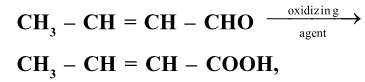the oxidizing agent can be

1. alkaline KMnO4

2. acidified K2Cr2O7

3. benedict's solution

4. All of the above

Concept Questions :-

Carboxylic Acids